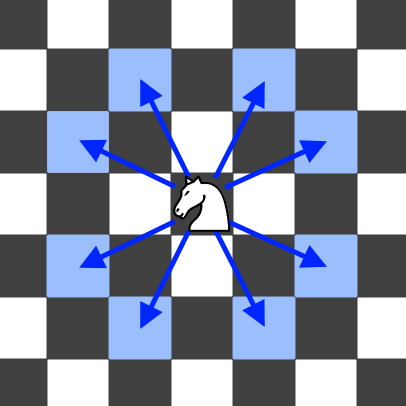##### Welcome to Subscribe On Youtube

Formatted question description: https://leetcode.ca/all/1197.html

# 1197. Minimum Knight Moves

Medium

## Description

In an infinite chess board with coordinates from -infinity to +infinity, you have a knight at square [0, 0].

A knight has 8 possible moves it can make, as illustrated below. Each move is two squares in a cardinal direction, then one square in an orthogonal direction.Return the minimum number of steps needed to move the knight to the square [x, y]. It is guaranteed the answer exists.

Example 1:

Input: x = 2, y = 1

Output: 1

Explanation: [0, 0] → [2, 1]

Example 2:

Input: x = 5, y = 5

Output: 4

Explanation: [0, 0] → [2, 1] → [4, 2] → [3, 4] → [5, 5]

Constraints:

• |x| + |y| <= 300

## Solution

According to the constraint, the size of the chess board is limited, so limit the chess board size to some smaller value. For example, suppose the chess board is 800 * 800, and the knight is initially at [400, 400]. The square [x, y] is adjusted to [x + 400, y + 400] accordingly.

Simply use breadth first search. A knight has at most 8 possible moves, so for each possible move, check whether the knight is still in the chess board after the move, and add the next square to the queue if it is still in the chess board. In this way, the search space can be reduced significantly.

Once the (updated) square [x, y] is reached, return the steps to reach the square, which is guaranteed to be the minimum number of steps.

• class Solution {
public int minKnightMoves(int x, int y) {
final int COORDINATE = 400;
x += COORDINATE;
y += COORDINATE;
final int WHITE = 0;
final int GRAY = 1;
final int BLACK = 2;
int length = COORDINATE * 2;
int[][] colors = new int[length][length];
int[][] distances = new int[length][length];
for (int i = 0; i < length; i++) {
for (int j = 0; j < length; j++)
distances[i][j] = Integer.MAX_VALUE;
}
colors[COORDINATE][COORDINATE] = GRAY;
distances[COORDINATE][COORDINATE] = 0;
queue.offer(new int[]{COORDINATE, COORDINATE});
int[][] directions = { {-2, -1}, {-2, 1}, {-1, 2}, {1, 2}, {2, 1}, {2, -1}, {1, -2}, {-1, -2} };
while (!queue.isEmpty()) {
int[] square = queue.poll();
int row = square, column = square;
int distance = distances[row][column];
for (int[] direction : directions) {
int newRow = row + direction, newColumn = column + direction;
if (newRow >= 0 && newRow < length && newColumn >= 0 && newColumn < length && colors[newRow][newColumn] == WHITE) {
colors[newRow][newColumn] = GRAY;
distances[newRow][newColumn] = distance + 1;
queue.offer(new int[]{newRow, newColumn});
}
}
colors[row][column] = BLACK;
if (colors[x][y] != WHITE)
return distances[x][y];
}
return -1;
}
}

• // OJ: https://leetcode.com/problems/minimum-knight-moves/
// Time: O(XY)
// Space: O(XY)
class Solution {
public:
int minKnightMoves(int x, int y) {
x = abs(x);
y = abs(y);
queue<pair<int, int>> q;
set<pair<int, int>> s;
q.emplace(0, 0);
s.emplace(0, 0);
int ans = 0, dirs = { {1, 2}, {1,-2}, {2,1},{2,-1},{-1,2},{-1,-2},{-2,1},{-2,-1} };
while (q.size()) {
int cnt = q.size();
while (cnt--) {
auto [a, b] = q.front();
q.pop();
if (a == x && b == y) return ans;
for (auto &[dx, dy] : dirs) {
pair<int, int> next = {a + dx, b + dy};
if (abs(next.first) + abs(next.second) > 300 || next.first < -1 || next.second < -1 || s.count(next)) continue;
s.insert(next);
q.push(next);
}
}
++ans;
}
return 0;
}
};

• class Solution:
def minKnightMoves(self, x: int, y: int) -> int:
q = deque([(0, 0)])
ans = 0
vis = {(0, 0)}
dirs = ((-2, 1), (-1, 2), (1, 2), (2, 1), (2, -1), (1, -2), (-1, -2), (-2, -1))
while q:
for _ in range(len(q)):
i, j = q.popleft()
if (i, j) == (x, y):
return ans
for a, b in dirs:
c, d = i + a, j + b
if (c, d) not in vis:
q.append((c, d))
ans += 1
return -1


• func minKnightMoves(x int, y int) int {
x, y = x+310, y+310
ans := 0
q := [][]int{ {310, 310} }
vis := make([][]bool, 700)
for i := range vis {
vis[i] = make([]bool, 700)
}
dirs := [][]int{ {-2, 1}, {-1, 2}, {1, 2}, {2, 1}, {2, -1}, {1, -2}, {-1, -2}, {-2, -1} }
for len(q) > 0 {
for k := len(q); k > 0; k-- {
p := q
q = q[1:]
if p == x && p == y {
return ans
}
for _, dir := range dirs {
c, d := p+dir, p+dir
if !vis[c][d] {
vis[c][d] = true
q = append(q, []int{c, d})
}
}
}
ans++
}
return -1
}